# DAV Class 6 Maths Chapter 6 Brain Teasers Solutions

The DAV Class 6 Maths Solutions and DAV Class 6 Maths Chapter 6 Brain Teasers Solutions of Introduction to Algebra offer comprehensive answers to textbook questions.

## DAV Class 6 Maths Ch 6 Brain Teasers Solutions

Question 1A.
(а) Mrs. Shalini is p years of age now, 3 years ago her age in years, was-
(i) 3 – p
(ii) 3 + p
(iii) p – 3
(iv) 3 + p
Solution:
(iii) p – 3
Present age of Mrs Shalini is = p years
Her age 3 years ago was (p – 3) years.
Hence, (iii) is the correct option.

(b) If the perimeter of a square is q metres, then length of each side in metres, is-
(i) q + 4
(ii) q ÷ 4
(iii) q – 4
(iv) 4 ÷ q
Solution:
(ii) q ÷ 4
Perimeter = 4 × side q = 4 × side
∴ side = q ÷ 4 metre
Hence, (ii) is the correct option.(c) Expression obtained when k is multiplied by 2 and then subtracted from 5, is-
(i) 2k – 5
(ii) 2k + 5
(iii) 5 – 2k
(iv) 5k – 2
Solution:
5 – 2k

(d) Number of notebooks bought for ₹ t at the rate of ₹ 40 per notebook, is-
(i) $$\frac{40}{t}$$
(ii) 40 t
(iii) $$\frac{t}{40}$$
(iv) t + 40
Solution:
(iii) $$\frac{t}{40}$$

(e) x = 6, y = 2, z = 3, what is the value of the expression $$\frac{x y-x z}{z^2}$$?
(i) $$\frac{-2}{3}$$
(ii) $$\frac{2}{3}$$
(iii) 3$$\frac{1}{3}$$
(iv) 5
Solution:
(i) $$\frac{-2}{3}$$
Put x = 6, y = 2, z = 3 in the expression $$\frac{x y-x z}{z^2}$$, we get
$$\frac{(6)(2)-(6)(3)}{(3)^2}$$
= $$\frac{12-18}{9}$$
= $$\frac{-6}{9}=-\frac{2}{3}$$(a) Your Mom has a sum of ₹ x. She spent ₹ 3000 on grocery and ₹ 2000 on milk. Write the algebraic expression for the amount left with her.
Solution:
₹ (x – 3000 – 2000) = (x – 5000)

(b) The digit in the ones place of a 2-digit number is twice the digit at the tens place. Write the number.
Solution:
T O
x 2x
Number = 10 × x + 1 × 2x
= 10x + 2x
= 12x

(c) If a notebook costs ₹ p and a pencil costs ₹ s, then what will be the cost of two notebooks and three pencils?
Solution:
Cost of 1 notebook = ₹ p
Cost of 2 notebooks = ₹ 2p …………(1)
Cost of 1 pencil = ₹ s
Cost of 3 pencils = ₹ 3x …(2)
On adding (1) and 2) we get
Cost of 2 notebooks + cost of 3 pencils = ₹ 2p + ₹ 3s
= ₹ (2p + 3s)

(d) What will you get if 3 times x is subtracted from the largest two-digit number?
Solution:
Largest 2-digit number = 99
3 times x = 3x
When 3 times x is subtracted from 99,
we get = 99 – 3x

(e) Rohit travels by car with the speed of x km/hr for 2 hours and 3 hours with the speed of y km/hr. Write the expression for total distance travelled by him.
Solution:
Distance = speed × time
Distance = x × 2 = 2x km and
Distance = y × 3 = 3y km
Total distance = (2x + 3y) kmQuestion 2.
Write the number which is
(a) 6 less than two-third of number x
(b) 3 times the sum of z and 5
Solution:
(a) 6 less than $$\frac{2}{3}$$ x = $$\frac{2}{3}$$ x – 6
(b) 3 times the sum of z and 5 = 3 (z + 5)

Question 3.
Write statements for:
(a) $$\frac{4}{5}$$ (a + 2)
(b) $$\frac{4}{5}$$ a + 2
Solution:
(a) $$\frac{4}{5}$$ (a + 2) = four-fifth the sum of 5 a and 2
(b) $$\frac{4}{5}$$ a + 2 = 2 more that four-fifth of 5 number a.

Question 4.
Write the following expressions using literals, numbers and arithmetic operations:
(a) diameter of a circle is twice its radius
(b) twice of x subtracted from one-third of y
(c) total cost of 3 tables at ₹ x each and 4 chairs at ₹ y each
(d) cost of one book if the cost of 10 books is ₹ x.
Solution:
(a) If d = diameter and
∴ d = 2r

(b) $$\frac{1}{3}$$ y – 2x

(c) ₹ (3x + 4y)

(d) ₹ $$\frac{x}{10}$$Question 5.
Write the product form of the following:
(a) – 3p3q2r
(b) (- y)27
Solution:
(a) – 3p3q2r
= – 3 × p × p × p × q × q × r

(b) (- y)21 = – y × – y × – y × ……….. 27 times

Question 6.
Write a statement to represent a constant.
Solution:
Number of paise in a rupee.

Question 7.
Write a statement to represent a variable.
Solution:
They are not same.

Question 8.
Write the product form of ($$\frac{2}{3}$$x)3 and $$\frac{2}{3}$$x3. Are they same?
Solution:
($$\frac{2}{3}$$x)3 = $$\frac{2}{3}$$x × $$\frac{2}{3}$$x × $$\frac{2}{3}$$
= $$\frac{8}{27}$$x3
$$\frac{2}{3}$$x3 = $$\frac{2}{3}$$ × x × x × x
= $$\frac{2}{3}$$x3
They are not same.Question 9.
Write one example each of a:
(а) Monomial expression
(b) Trinomial expression
(d) Binomial expression
Solution:
(a) 3x
(b) 2a + b + 3
(c) a + b + c + 5
(d) 2x + 3y

Question 10.
Separate the terms of:
3p3 – 2p2 + p – 6.
Solution:
3p3 – 2p2, p, – 6

Question 11.
Encircle the like terms:
a2bc, a2b2c, b2a2c2, – ba2c, c2ab, bca2, cba2 – a2bc.
Solution:
a2bc, – ba2c, – a2bc, bca2, cba2Question 12.
Fill in the blanks:
(a) An algebraic expression whose terms are – 2a, 3b, – 5 is ________.
Solution:
– 2a + 3b – 5

(b) The numerical coefficient in is ________.
Solution:
– $$\frac{3}{2}$$

(c) The coefficient of y in 2.5 x2y is ________.
Solution:
2.5 x2

(d) The coefficient of p in – p is ________.
Solution:
– 1

(e) Is 2x – 3y – x a binomial or a trinomial expression?
Solution:
binomial

(f) The exponential form of (- p) × (- p) × (- p) × …………. 17 times is ________.
Solution:
(- p)17

(g) Sum of – 7x, 8x, x, – 9x is ________.
Solution:
– 7x

(h) If we subtract – 9y from – 2y, we get ________.
Solution:
7yQuestion 13.
Evaluate a – 4b + 2 if a = 2, b = 1.
Solution:
Here a = 2, b = 1
a – 4b + 2 = 2 – 4(1) + 2
= 4 – 4 = 0

Question 14.
In a bus x passengers have taken, tickets of ₹ 5 each and y passengers have taken tickets of ₹ 10 each. Find the total number of passengers and total fare received by the conductor.
Solution:
Fare of x passengers = ₹ 5x
Fare of y passengers = ₹ 10y
Total fare = ₹ 5x + ₹ 10y = ₹ (5x + 10y)
Total number of passengers = x + y.

Question 15.
On a particular day x ladies were travelling in ladies compartment of metro rail and y people in other compartments from Station A to Station B. If 10 passengers from other compartments and 15 passengers (ladies) from ladies compartment got down in between, write the algebraic expression for the number of passengers left at Station B.
Ans.
No. of people travelling in other compartments = y
∴ Total no. passengers = (x + y)
No. of ladies got down =15
No. of people got down = 10
∴ Total number of passengers got down = 15 + 10 = 25
∴ No. of passengers left = Total no. of passengers – Total no. of passengers got down
= (x + y) – 25.### DAV Class 8 Maths Chapter 1 HOTS

Question 1.
If two cubes whose volumes are given by (x3 + 6y2 + 12z + 8) cubic units and (x3 – 2z + 10 – y2) cubic units respectively are melted to form a new cube, find the expression representing the volume of the new cube thus formed.
Solution:
Vol. of first cube = (x3 + 6y2 + 12z + 8) cubic units
Vol. of second cube = (x3 – 2z + 10 – y2) cubic units
∴ Volume of new cube = Vol. of first cube + Vol. of second cube
= (x3 + 6y2 + 12z + 8) + (x2 – 2z + 10 – y2)
= (x3 + x3) + (6y2 – y2) + (12z – 2z) + (8 + 10)
= 2x3 + 5y2 + 10z + 18 cubic unitsQuestion 1.
Identify the like terms from the following:
a2b, – ba2, 3a2b2, 7ab2, – a2b2, 7a2b
Solution:
Like terms are a2b, 7a2b.

Question 2.
Fill in the blanks:

(a) 2p + 3q = ____ + 2p
(b) x + 2y = ____ + x
(c) 2x × ____ = 2x
(d) 6x + ____ = 6x
Solution:
(a) 3q
(b) 2y
(c) 1
(d) 0

Question 3.
Write the following in exponential form:
(a) (2x) × (2x) × (2x) …………… 11 times
(b) $$\left(-\frac{1}{2} x\right) \times\left(-\frac{1}{2} x\right) \times\left(-\frac{1}{2} x\right)$$
(c) $$\left(\frac{3 y}{2}\right) \times\left(\frac{3}{2} y\right) \times\left(\frac{3}{2} y\right)$$
(d) x × x × x × x × x × x
Solution:
(a) (2x) × (2x) × (2x) × ……………. 11 times
= (2x)11
(b) $$\left(-\frac{1}{2} x\right) \times\left(-\frac{1}{2} x\right) \times\left(-\frac{1}{2} x\right)=\left(-\frac{1}{2} x\right)^3$$
(c) $$\left(\frac{3 y}{2}\right) \times\left(\frac{3}{2} y\right) \times\left(\frac{3}{2} y\right)=\left(\frac{3}{2} y\right)^3$$
(d) x × x × x × x × x × x = (x)6Question 4.
Write the product form of the following:
(a) $$\left(-\frac{2}{7}\right)^{23}$$
(b) $$\left(\frac{5}{6} x y\right)^3$$
(c) (- 2x)7
(d)$$\left(\frac{1}{7} x^2 y z\right)^3$$
Solution:
(a) ($$\frac{- 2}{7}$$)23
= $$\frac{- 2}{7}$$ × $$\frac{- 2}{7}$$ × $$\frac{- 2}{7}$$ ……………. 23 times

(b) ($$\frac{5}{6}$$ xy)3
= $$\frac{5}{6}$$ xy × $$\frac{5}{6}$$ xy × $$\frac{5}{6}$$ xy

(e) (- 2x)7 = (- 2x) × (- 2x) × (- 2x) × (- 2x) × (- 2x) × (- 2x) × (- 2x)
(d) ($$\frac{1}{7}$$ x2yz)3
= $$\frac{1}{7}$$ x2yz × $$\frac{1}{7}$$ x2yz × $$\frac{1}{7}$$ x2yz

Question 5.
Identify the statements as constants and variables.

(a) Two and two make four
Solution:
Constant

(b) Temperature in a day
Solution:
Variable

(c) Number of days in a year
Solution:
Variable

(d) Number of days in a leap year
Solution:
Constant

(e) Number of students in a school
Solution:
Variable

(f) Number of players in a cricket team
Solution:
Constant

(g) Number of books in a library
Solution:
Variable

(h) Number of workers in a factory
Solution:
VariableQuestion 6.
Write the numerical coefficients in the following terms:

(a) – $$\frac{2}{3}$$ y
Solution:
– $$\frac{2}{3}$$

(b) $$\frac{5}{6}$$ x
Solution:
$$\frac{5}{6}$$

(c) $$\frac{1}{2}$$ xy2
Solution:
$$\frac{1}{2}$$

(d) – 2y
Solution:
– 2

(e) – p
Solution:
– 1

(f) 8x2y
Solution:
8

(g) – 2yz2
Solution:
– 2

(h) – 3m
Solution:
– 3

(i) 2n
Solution:
2Question 7.
Find the monomial, binomial, trinomial and quadrinomial terms.
3, a + b, 3x + 2y, a + b – 2c, x + 2y – y2 + 7, 0, 5yz2, 3x + 7y, – 3 + 2y + 3z
Solution:
Monomial = 3, 0, 5yz2
Binomials = a + b, 3x + 2y, 3x + 7y
Trinomials = a + b – 2e, – 3 + 2y + 3z
Quadrinomials = x + 2y – y2 + 7

Question 8.
Add the following using column method:
(a) x3 + y3 + 3x2y, 3x3 – y3 + 2x2y and 6x3 + y3 – 3x2y
(b) – p – q, 3p + q and p + 3q
(c) 5x2 – y2 – 8z and 3x2 +y2 + 8z
(d) x + y – z and – x – y + z
Solution:
(a) x3 + y3 + 3x2y, 3x3 – y3 + 2x2y and 6x3 + y3 – 3x2y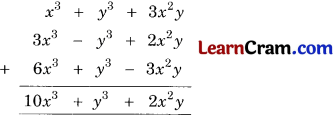(b) – p – q, 3p + q and p + 3q(c) 5x2 – y2 – 8z and 3x2 +y2 + 8z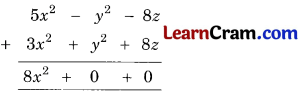= 8x2

(d) x + y – z and – x – y + z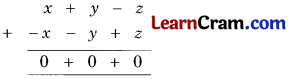= 0Question 9.
Subtract: 8x3 + 3x2 + 6x – 5 from x3 – 5x2 + 8x + 9.
Solution: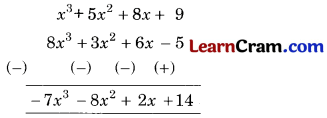Question 10.
From the sum of 7x3 + 2x + 7 and x3 + 8x2 – 3 subtract x3 – x2 – x + 5.
Solution:
sum of 7x3 + 2x + 7 and x3 + 8x2 – 3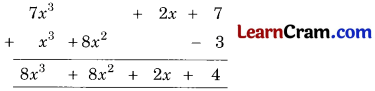Now we have to subtract x3 – x2 – x + 5 from 8x3 + 8x2 + 2x + 4